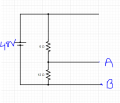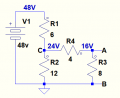# How to calculate the Thevenin Voltage and Thevenin Resistance between the terminal A and B of the follwing figure

#### studyhard24

Joined Jun 20, 2022
35

#### ericgibbs

Joined Jan 29, 2010
18,211
hi 24,
Welcome to AAC.
As this is homework you must post your best attempt at answering, we can the help.
E

#### studyhard24

Joined Jun 20, 2022
35As the 8 Ohm is parallel to the Open circuit with A and B so removing it doesn't affect the equivalent voltage.
After removing it,
we can also remove the 4 Ohm resistance because the current across is equal to the open circuit lane.

After doing so, I'm stuck. What should I do with the upper open portion, should I make the 6 Ohm and 12 Ohm in parallel or in series. I'm new to this course, and so I lack some knowledge. Not able to figure out

#### crutschow

Joined Mar 14, 2008
33,317
Do you know how to calculate the equivalent value of series and parallel resistances?
You need that to determine the equivalent circuit Thevenin resistance.

#### crutschow

Joined Mar 14, 2008
33,317
As the 8 Ohm is parallel to the Open circuit with A and B so removing it doesn't affect the equivalent voltage.
But it does.
If the resistor has current flowing through it then it affects the equivalent voltage.
Why do you think it doesn't?

#### studyhard24

Joined Jun 20, 2022
35
But it does.
Why do you think it doesn't?
as the voltage across two resistor which are parallel is the same. Please correct me if I am wrong

#### crutschow

Joined Mar 14, 2008
33,317
as the voltage across two resistor which are parallel is the same. Please correct me if I am wrong
That is true.
But why do you think that means you can remove the resistors?

#### studyhard24

Joined Jun 20, 2022
35
That is true.
But why do you think that means you can remove the resistors?
Pardon my mistake, so we can't remove any resistor then. I know how to calculate equivalent resistance. I'm confused if I need to do anything for the open circuit along with the 6 Ohm resistor.

#### crutschow

Joined Mar 14, 2008
33,317
I'm confused if I need to do anything for the open circuit along with the 6 Ohm resistor.
Okay, you need to calculate the equivalent resistance of the 4, 8, and 12 ohm resistors from the viewpoint of the terminal connected to the 6 ohm resistor.
The 6 ohm resistor is in series with that equivalent resistance, allowing you to calculate the divider voltage at that junction.

From that you can calculate the equivalent output voltage due to the 4Ω and 8Ω divider.

•studyhard24

#### studyhard24

Joined Jun 20, 2022
35
So I did it. Finally

#### studyhard24

Joined Jun 20, 2022
35
Okay, you need to calculate the equivalent resistance of the 4, 8, and 12 ohm resistors from the viewpoint of the terminal connected to the 6 ohm resistor.
The 6 ohm resistor is in series with that equivalent resistance, allowing you to calculate the divider voltage at that junction.

From that you can calculate the equivalent output voltage due to the 4Ω and 8Ω divider.
Mr. crutschow Sir. please check. Thank you, really appreciated

#### crutschow

Joined Mar 14, 2008
33,317
Below is a simulation of the voltages:

And how can the Thevenin resistance between A and B be greater than 9Ω when R3 is 8Ω?
The first rule after getting a solution to a problem is to see if your answer makes sense or is not obviously incorrect.

So you need to revisit your calculations for both voltage and resistance.

One way to do it---

Voltage calculation-
1. Calculate the equivalent impedance or R2, R4, and R3, from Node C to node B (ignoring R1).
2. From that, calculate the voltage at node C using the value for R1 and that equivalent impedance.
3. From that, calculate the voltage at Node A due to the R3 and R4 divider.
Thevenin output impedance-
1. Calculate the equivalent parallel impedance of R1 and R2 at point C to point B (remember V1 has zero impedance).
2. From that, calculate the impedance from point A to point B.
Note that none of the calculations give fractional answers.Last edited:

#### MrAl

Joined Jun 17, 2014
10,871
So I did it. Finally
Just a quick note here.

Do you know how to use Nodal Analysis or any other type of network analysis?
I ask because you can check your own work if you do.

Step 1: You start by simply adding a parallel resistance across the output A and B. You then compute the output voltage.
Step 2: You compute your Thevenin or Norton equivalent circuit, then add the same parallel resistance across A and B (to the new network). You then compute the output voltage once again.
Step 3: Compare results from Step 1 and Step 2. If they are the same, you have found the Thevenin or Norton values correctly. If they are different, you made a mistake somewhere.

Note the parallel resistance could be symbolic, or if an actual resistor value then try to choose one that is comparable to the other resistances. In your circuit that would mean maybe 8 Ohms or 12 Ohms or something like that, but not really 1000 Ohms or 10000 Ohms because that is way different than the resistor values in the circuit to start with. You dont have to worry about this really but if you choose the parallel resistance right if there is an error it shows up as a bigger difference if you make a mistake, you dont want to have to think about a difference of 1 microvolt with an output of 100 volts for example.
If you do the calculations symbolically it is even better as the error, if any, will show up as a different expression altogether.

Being able to check your own work is a HUGE part of engineering. There will be many times when there isnt anyone else around to ask, and other times when you are the only one that knows how to do it at all as the rest of the staff may be trained in some other discipline.